## Building a 16-bit PWM DAC out of 8-bit Fractional PWM and DeltaSigma ModulatorLevel 9
Level 9# Building a 16-bit PWM DAC out of 8-bit Fractional PWM and DeltaSigma Modulator

Hi,

Uploaded below is a demo project showing 16-bit PWM DAC using 8-bit Fractional PWM and 8-bit Delta-Sigma Modulator and simple RC-filter.

The PWM DACs are popular in budget audio applications due to their simplicity. The known issue of this approach is very slow response due to the necessity to filter out the main PWM harmonic: PWM_Clock / 2^N, where N - is the number of PWM bits.  For 16-bit PWM, and clock frequency of 48MHz, this results in 48MHz / 2^16 =  732 Hz, which is  rather slow for audio use. For 8-bit PWM, the main lobe is located at 48MHz / 2^8 =  187.5 kHz, which is acceptable for audio applications. Unfortunately, 8-bit output amplitude is not nearly enough in most cases. The project below tries to resolve this dilemma by using fast 8-bit Fractional PWM, where output duty cycle is externally switched between the (MSB) and (MSB+1) positions using custom Delta-Sigma Modulator. The PWM provides the MSB (most significant bit), while Delta-Sigma Modulator provides the LSB. This way the PWM can operate at high frequency of 187.5kHz, while the DeltaSigma Modulator provides extra bits of resolution.

As usual with PWM DACs, the absolute accuracy depends on the stability of the driving voltage Vdd, output buffer speed and symmetry against Vdd and Vss (Gnd). Any fluctuations on the power rail directly affect the DAC output. Simple RC filter provides -43dB suppression of the PWM main harmonic. Using (pseudo-) second-order filter, made of two RC filters in series, suppresses it below -75dB.

Attached are two projects: basic and extended. The basic project includes all necessary components, and simply outputs a single voltage. The extended project scans the output from 0-65535, and uses ADC for sampling the DAC values and displaying them using SerialPlot charting software.

/odissey1

Projects include modified 1-st-order Delta-Sigma Modulator, originally developed by FY_ZHU

Extended project also uses additional custom components:

SerialPlot v0.0: Interface to real-time data charts

PSoC Annotation Library v1.0 (optional)

Figure1. Fractional PWM DAC using 8-bit PWM modulated by 1-st order 8-bit DeltaSigma Modulator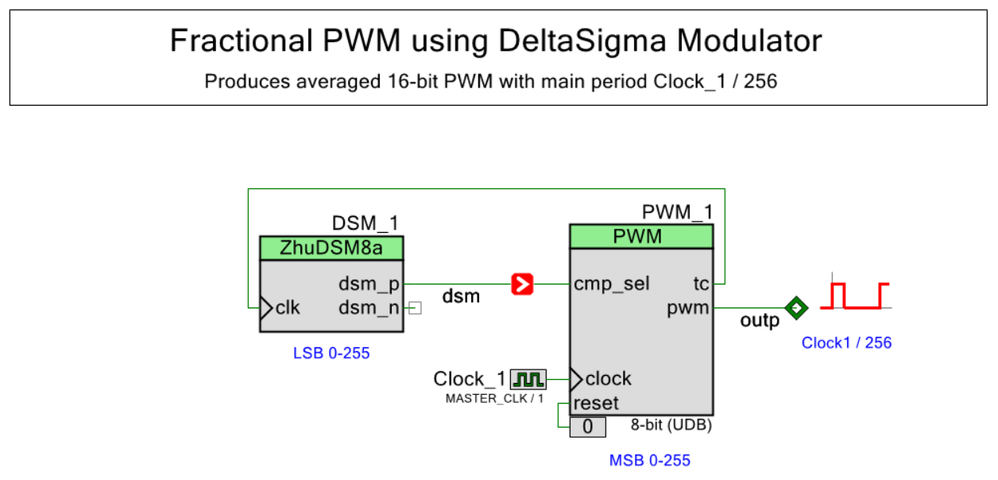Figure 2. PWM output is filtered by simple 1-st order RC filter with R1=2.2k, C1=100nF.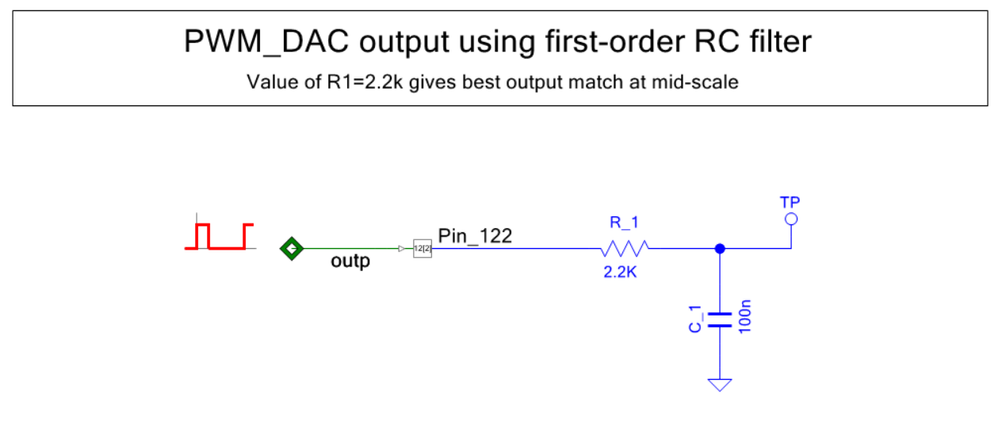Figure 3. Project annotation using PSoC Annotation Library v1.0. The ADC serves as a voltmeter. The physical connection between Pin 12.7 (UART TX) and the SerialPlot charting software is not required.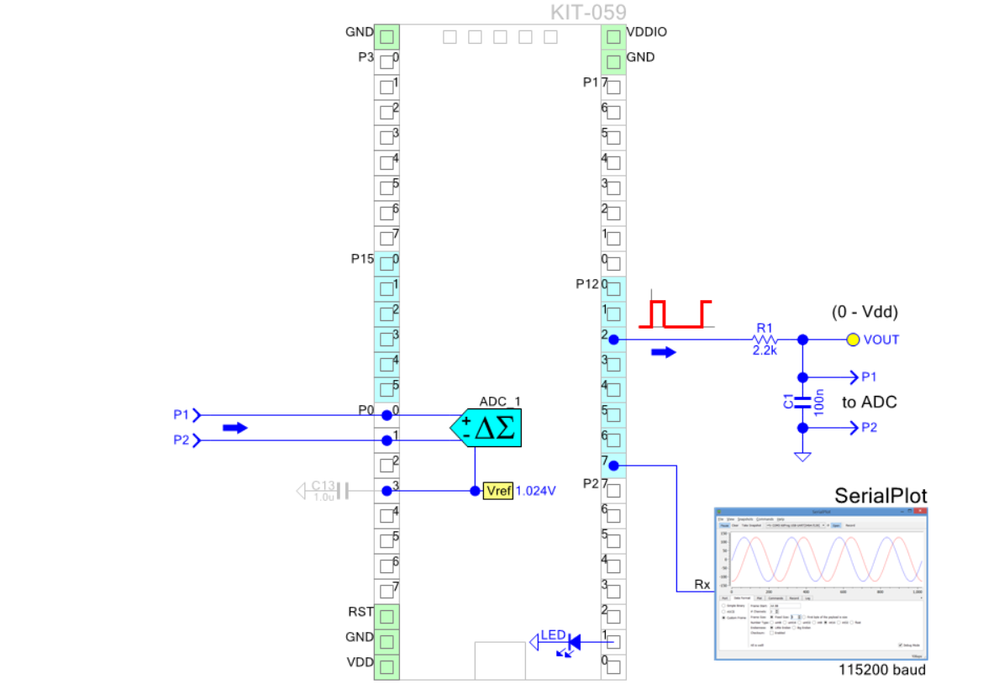Figure 4. SerialPlot output while scanning DAC code 0 to 65500. Blue -  DAC code, Red  - result measured by ADC, Green - residual (ADC sample - DAC Code). R1=2.2k.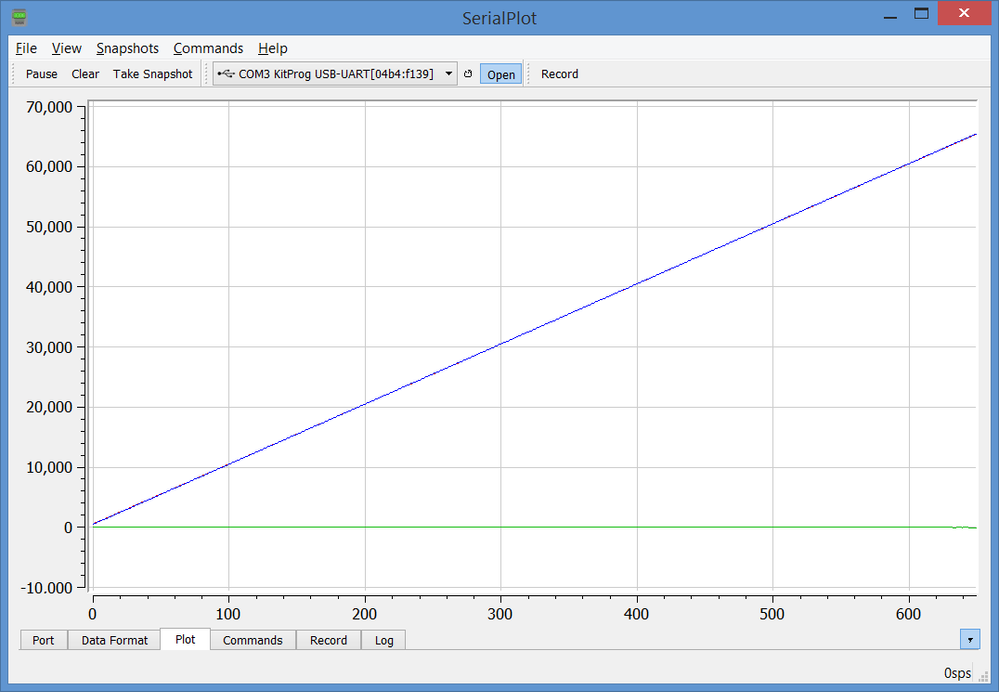Figure 5. SerialPlot output of the residual (ADC sample - DAC code)  for R1=2.2k. Note that the shape of the curve varies with R1 value. The noise is due to the Vdd instability of the USB-powered PSoC prototyping board.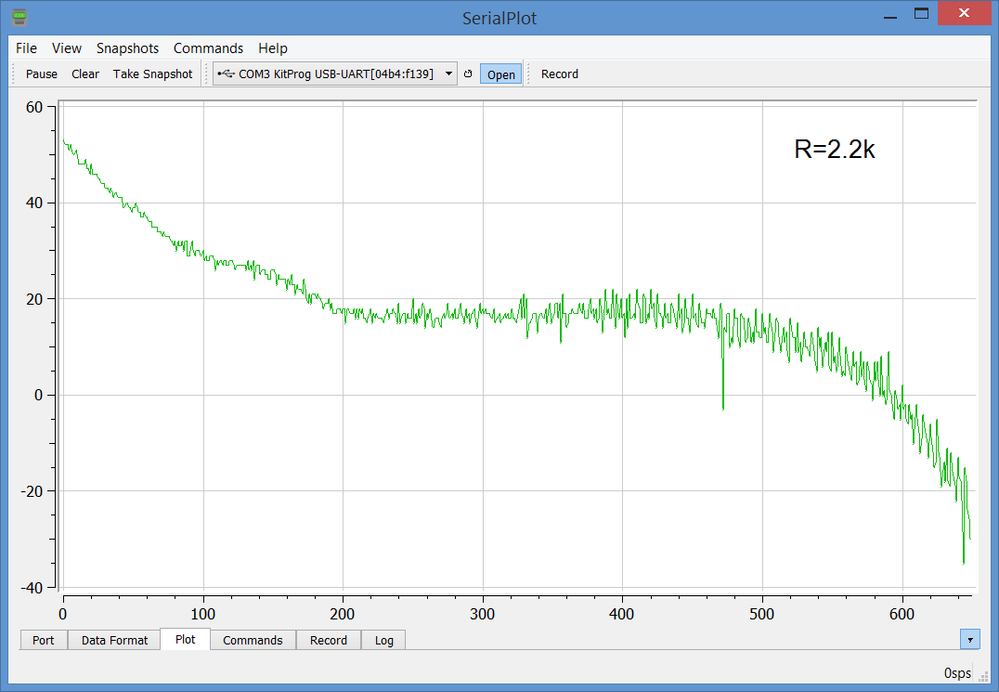Figure 6. SerialPlot output of the residual (ADC sample - DAC code)  for R1=1.0k.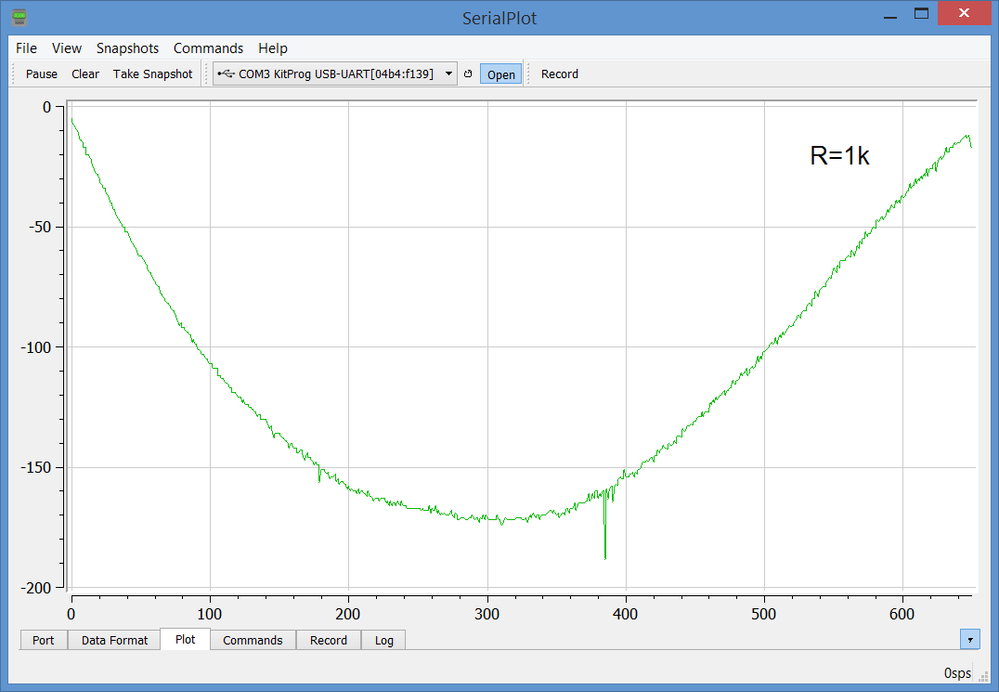Figure 7. SerialPlot output of the residual (ADC sample - DAC code)  for R1=4.7k.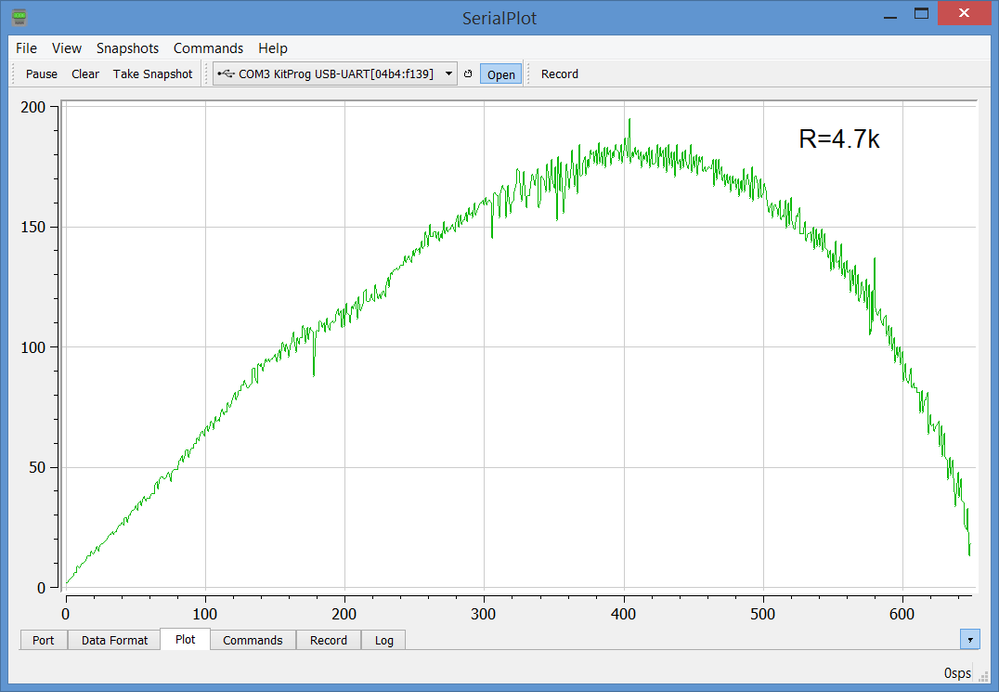Figure 8. FFT of the AC-coupled DAC output at mid-scale (DAC code = 32767), after single RC filter (R1=2.2k, C1=100nF). The PWM at 187.5 kHz p-p is ~40mV (-43dB). The noise floor is at about -75dB.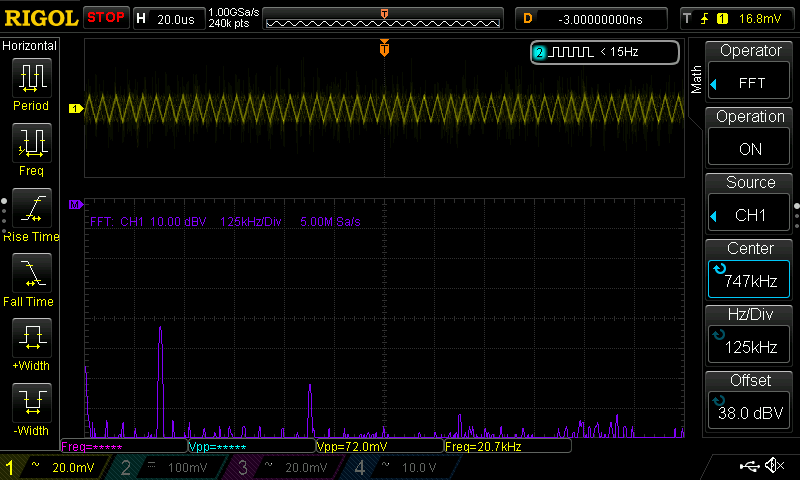Figure 9. FFT of the AC-coupled DAC output at mid-scale (DAC code = 32767), filtered using double serial filter R1C1-R2C2 (R1=2.2k, C1=100nF, R2=2.2k, C2=100nF). The PWM noise at 187.5 kHz is not discernible above the noise floor (-75dB).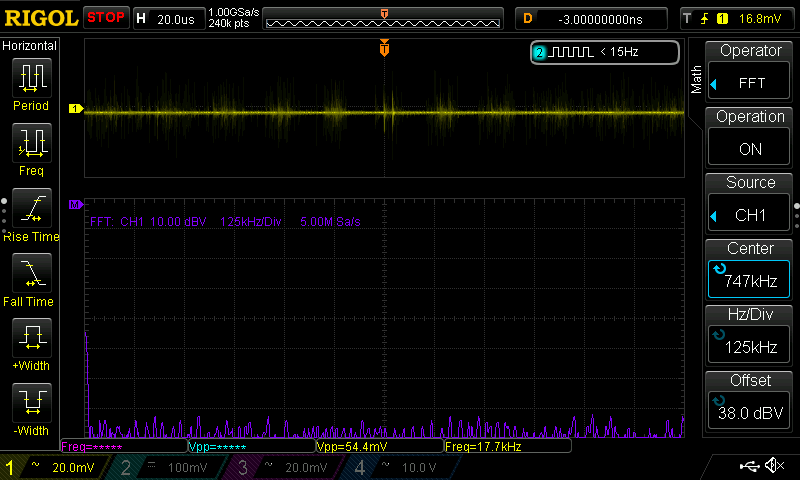ModeratorModerator# Re: Building a 16-bit PWM DAC out of 8-bit Fractional PWM and DeltaSigma Modulator

Hi,

Thank you for the contribution.

Regards

Alakananda